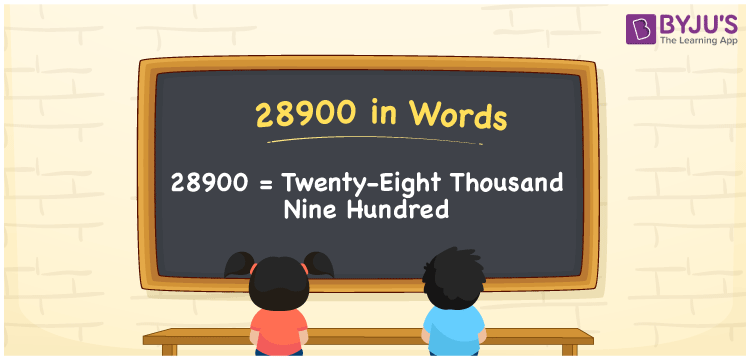# 28900 in Words

28900 in words is twenty-eight thousand nine hundred. If the cost of the two-wheeler is Rs. 28900, it can be written as “The cost of the two-wheeler is Twenty-eight thousand nine hundred rupees”. Hence, to express the value of the object, the knowledge of numbers and the number names is essential. This article will discuss the procedure of writing the number 28900 in words using the place value chart in detail.

 28900 in Words: Twenty-eight Thousand Nine Hundred. Twenty-eight Thousand Nine Hundred in Numerical Form: 28900.

## 28900 in English Words## How to Write 28900 in Words?

The place values of the 5-digit number 28900 are presented below.

 Ten-thousands Thousands Hundreds Tens Ones 2 8 9 0 0

The expanded form of 28900 is as follows:

= 2 × Ten thousand + 8 × Thousand + 9 × Hundred + 0 × Ten + 0 × One

= 2 × 10000 + 8 × 1000 + 9 × 100 + 0 × 10 + 0 × 1

= 20000 + 8000 + 900

= 28900

= twenty-eight thousand nine hundred

Hence, 28900 in words is twenty-eight thousand nine hundred.

28900 in words – Twenty-eight thousand nine hundred

Is 28900 an odd number? – No

Is 28900 an even number? – Yes

Is 28900 a perfect square number? – Yes

Is 28900 a perfect cube number? – No

Is 28900 a prime number? – No

Is 28900 a composite number? – Yes

## Frequently Asked Questions on 28900 in Words

Q1

### Write 28900 in words.

28900 in words is twenty-eight thousand nine hundred.

Q2

### Simplify 28000 + 900, and express it in words.

Simplifying 28000 + 900, we get 28900. Hence, 28900 in words is twenty-eight thousand nine hundred.

Q3

### Is 28900 a perfect square number?

Yes, 28900 is a perfect square number.